# KVPY-SA 2016 Mathematics Paper with Solutions

In this section, we have provided the most comprehensive and accurate KVPY Maths 2016 paper’s solutions. This page includes answers to all the questions of KVPY SA 2016 Maths paper. Previous years’ papers are the best study material students can have to study the different concepts asked in the exam. Students can download these solutions in PDF format for free and the link is provided at the bottom of this page.
Few important topics covered in this paper are: Polynomial, Quadrilateral, Circle, Sets, Quadratic equation and Probability.

### KVPY SA 2016 - Maths

Question 1: Suppose the quadratic polynomial P(x) = ax2 + bx + c has positive coefficients a, b, c in arithmetic progression in that order. If P(x) = 0 has integer roots α and β, then α + β + αβ equals

1. a. 3
2. b. 5
3. c. 7
4. d. 14

Solution:

Given, P(x) = ax2 + bx + c has integral roots α, β and a, b, c are in AP.

Now, α + β + αβ = -b/a + c/a = (c-b)/a = (b-a)/a

α + β + αβ = b/a – 1 ∈ I (As α and β are integers)

b/a ∈ I

b = λ a and c = 2(2λ – 1) …(1)

Again, Since P(x) = 0 has integral roots, therefore its discriminant must be a perfect square.

i.e. D = b2 - 4ac

= a22 – 4(2 λ – 1)) using (1)

So, λ2 - 8 λ + 4 = k2, λ ∈ I …(2)

Again, discriminant of above equation must be perfect square λ ∈ I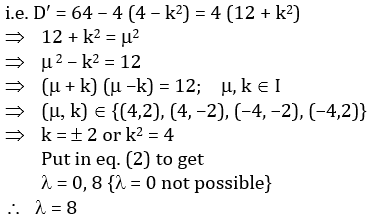Thus, P(x) = ax2 + 8a λ + 15a = 0

a (x2 + 8x + 15) = 0

a(x + 3) (x + 5) = 0

x = –3,–5

α + β + αβ = – 8 + 15 = 7

Question 2: The number of digits in the decimal expansion of 165516 is

1. a. 16
2. b. 17
3. c. 18
4. d. 19

Solution:

The given number is,

165. 516 = (24)5. 516

= 24. 216. 516

= 16.1016

It is an eighteenth digit number with 16 zeroes.

Question 3: Let t be real number such that t2 = at + b for some positive integers a and b. Then for any choice of positive integers a and b, t3 is never equal to

1. a. 4t + 3
2. b. 8t + 5
3. c. 10t + 3
4. d. 6t + 5

Solution:

Given, t2 = at + b, a b ∈ z+

Multiplying both sides by t

t3 = at2 + bt

= a (at + b) + bt

= (a2 +b) t + ab ……. (1)

Now

(A) If t3 = 4t + 3

Comparing with eq. (1),

a2 + b = 4, ab = 3

a = 1, b = 3 (Possible)

(B) If t3 = 8t + 5

Comparing with eq. (1),

a2 + b = 8, ab = 5

No Integral Values of a, b (Hence, not possible.)

(C) If t3 = 10t + 3

Comparing with eq. (1),

a2 + b = 10, ab = 3

a = 3, b = 1 (Possible)

(D) If t3 = 6t + 3

Comparing with eq. (1),

a2 + b = 6, ab = 1

a = 1, b = 5 (Possible)

Question 4: Consider the equation (1+ a + b)2 = 3(1+ a2 + b2), where a, b are real numbers. Then

1. a. There is no solution pair (a, b).
2. b. There are infinitely many solution pairs (a, b).
3. c. There are exactly two solution pairs (a, b).
4. d. There is exactly one solution pair (a, b).

Solution:

Given, (1 + a + b)2 = 3 (1 + a2 + b2)

1 + a2 + b2 + 2(a+ b + ab) = 3 (1+ a2 + b2)

2(a2 + b2 + 1 – a – b – ab) = 0

(a – 1) 2 + (b – 1) 2 + (a – b) 2 = 0

a – 1 = b – 1 = a – b = 0

a = b = 1

Thus, (a, b) = (1, 1) is only possible solution.

Question 5: Let a1, a2, ........, a100 be non-zero real numbers such that a1 + a2 + ....... +a100 = 0, Then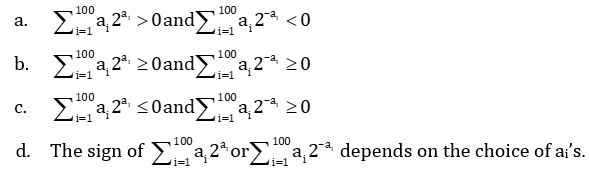Solution: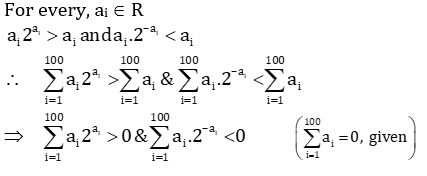Question 6: Let ABCD be a trapezium in which AB is parallel to CD, AB = 11, BC = 4, CD = 6 and DA = 3. The distance between AB and CD is

1. a. 2
2. b. 2.4
3. c. 2.8
4. d. Not determinable with the data

Solution: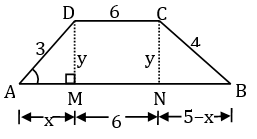In ΔAMD, using Pythagoras Theorem,

x2 + y2 = 32 …… (1)

Again, in ΔCNB, using Pythagoras Theorem,

(5 - x) 2 + y2 = 42 …… (2)

Subtracting eq.(2) from eq. (1),

x2 – (5 – x) 2 = 32 – 42

x = 9/5

Therefore, y = 2.4

Question 7: The point A, B, C, D, E are marked on the circumference of a circle in clockwise direction such that ∠ABC =130o and ∠CDE = 110o. The measure of ∠ACE in degrees is

1. a. 50°
2. b. 60°
3. c. 70°
4. d. 80°

Solution: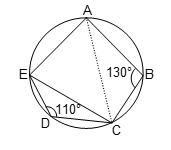∠CDE + ∠CAE = 180° {Sum of opposite angles of a cyclic quadrilateral is 180°.}

110° + ∠ CAE = 180°

∠CAE = 70°…… (1)

∠ABC+ ∠ AEC = 180° {Sum of opposite angles of a cyclic quadrilateral is 180°.}

∠AEC = 50°……. (2)

Now, in ΔACE,

∠ACE + ∠CEA + ∠EAC = 180° {Sum of angles of a triangle is 180°.}

∠ ACE + 50° + 70° = 180°

∠ACE = 60°

Question 8: The circle of radii 1, 2 and 3 units respectively touch each other externally in the plane. The circumradius of the triangle formed by joining the centers of the circles is

1. a. 1.5
2. b. 2
3. c. 2.5
4. d. 3

Solution: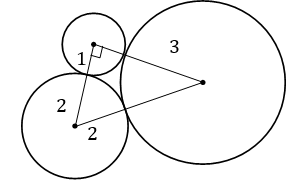The triangle so formed joining centres of three circles is right angled triangle, with side lengths 3, 4 and 5.

Circumradius = 5/2 = 2.5 {In a right triangle hypotenuse is diameter}

Question 9: Let, P be a point inside a triangle ABC with ∠ABC = 90°. Let, P1 and P2 be the images of P under reflection in AB and BC respectively. The distance between the circumcenters of triangles ABC and P1 P P2 is

1. a. AB/2
2. b. AC/2
3. c. (AP + BP + CP)/3
4. d. (AB + BC + AC)/2

Solution:

1.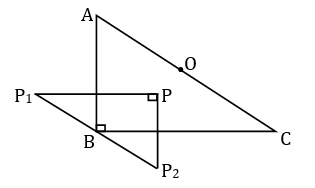Since, P1 is image of P in line mirror AB, therefore AB is perpendicular bisectors of PP1.

Similarly, CB is perpendicular bisector of PP2.

Therefore, B is Circum-Centre of ΔPP1P2.

{The point of intersection of perpendicular bisectors}

Also, Circum-Centre of ΔABC is O i.e. midpoint of hypotenuse, since it is a right angled triangle.

Thus, required distance = OB (Circum radius of ΔABC) = AC/2.

Question 10: Let a and b be two positive real numbers such that a + 2b ≤ 1. Let A1 and A2 be, respectively, the areas of circles with radii ab3 and b2. Then the maximum possible value of A1/A2 is

1. a. 1/16
2. b. 1/64
3. c. 1/16√2
4. d. 1/32

Solution: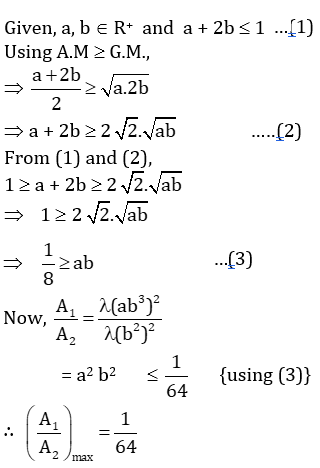Question 11: There are two candles of same length and same size. Both of them burn at uniform rate. The first one burns in 5 hours and the second one burns in 3 hours. Both the candles are lit together. After how many minutes the length of the first candle is 3 times that of the other?

1. a. 90
2. b. 120
3. c. 135
4. d. 150

Solution:

Let ‘l’ be the initial length of two candles and r1 and r2 their respective rates of burning.

r1 = l/5 and r2 = l/3

Now, if after time ‘t’, their lengths are l1 and l2.

Then, l1 = l – l/5 x t and l2 = l - l/5 x t ………..(1)

Also, l1 = 3 l2 (given)

l - (l/5)t = 3 (l– l/5 x t) {Using eq.(1)}

t - t/5 = 2

t = 2.5 hours=150 minutes

Question 12: Consider a cuboid all of whose edges are integers and whose base is square. Suppose the sum of all its edges are numerically equal to the sum of the areas of all its six faces. Then the sum of all its edges is

1. a. 12
2. b. 18
3. c. 24
4. d. 36

Solution:

Let, the side of square base be ‘a’ and height of box be ‘b’, where a, b ∈ I+

Given, Sum of Edges = Sum of areas of Six faces

i.e. 4a + 4a + 4b = 2 (a2 + ab + ab)

a2 - 4a + 2b (a - 1) = 0

2b = (a2 - 4a)/(1-a) < 0

{because b ∈ I+}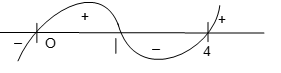a ∈ (0, 1) U (1,4)

Therefore, possible integral values of ‘a’ are 2 and 3.

If a = 2, b = 2 and

If a = 3, b = 3/4 ∉ z+ ; rejected

Therefore, Edges are 2,2,3

Thus, Sum of all edges = 4 (2 + 2 + 3) = 24

Question 13: Let, A1, A2,...., Am be non-empty subsets of {1,2,3,..... ,100} satisfying the following conditions:

(1) The numbers |A1|, |A2|,... ,|Am| are distinct.

(2) A1, A2, ... , Am are pairwise disjoint.

(Here, |A| denotes the number of elements in the set A. Then the maximum possible value of m is

1. a. 13
2. b. 14
3. c. 15
4. d. 16

Solution:

Each subset A1, A2,...., Am has distinct no. of elements and are pair-wise disjoint

Therefore, for maximum value of ‘m’

|A1| = 1, |A2| = 2, ……, |Am| = m

Also, 1 + 2 +3 + ……. + m ≤ 100

So, m(m+1)/2 ≤ 100

m < 14

Therefore, m = 13

Question 14: The number of all 2-digit numbers n such that n is equal to the sum of the square of digit in its tens place and the cube of the digit in units place is

1. a. 0
2. b. 1
3. c. 2
4. d. 4

Solution:

Let, n = xy (Where x is tens place digit and y is units’ place digit)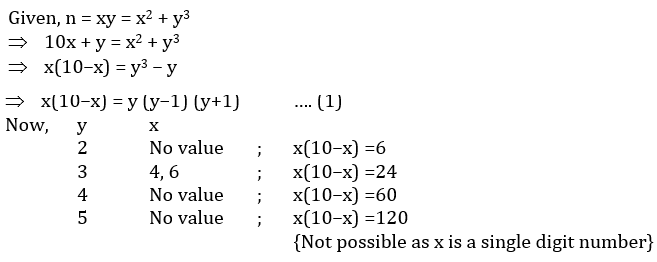Therefore, The possible numbers are 43 and 63.

Question 15: Let f be a function defined on the set of all positive integers such that f(xy) = f (x) + f (y) for all positive integers x, y. If f (12) = 24 and f (8) = 15, the value of f (48) is

1. a. 31
2. b. 32
3. c. 33
4. d. 34

Solution:

Given, f(xy) = f(x) +f(y) for all x, y ∈ I+ …. (1) and f (12) = 24 and f (8) = 15

Now, put x = 4, y = 2 in eq. (1)

f(8) = f(4) + f(2) ……..(2)

put x = 2, y = 2 in eq. (1)

f(4) = f(2) + f(2) = 2f(2) ……..(3)

From (2) & (3)

f(8) = 3f(2)

3f(2) = 15 {as, f (8) = 15}

f(2) = 5, f(4) = 10

Now, f(48) = f(12 × 4) = f(12) + f(4)

f(48) = 24 + 10 {∵ f (12) = 24}

f(48) = 34

Question 16: Suppose a is a positive real number such that a5 – a3 + a = 2. Then

1. a. a6 < 2
2. b. 2 < a6 < 3
3. c. 3 < a6 < 4
4. d. 4 ≤ a6

Solution:

Given, a5 – a3 + a – 2 = 0

a5 – a3 = 2 – a …… (1)

a5 = a3 – a + 2

a7 = a5 – a3 + 2a2 {multiplying by a2}

a7 =2 - a + 2a2 {using eq. (1)}

a6 = 2(a + 1/a ) – 1 …….(2)

Now, let f(a) = a6 and g(a)= 2(a + 1/a) – 1

f(1) = 1; g(1) = 3 and f(2) = 64, g(2) = 4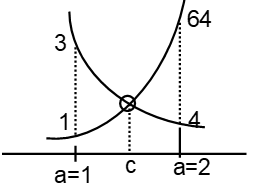Since, f(1) < g(1) and f(2) > g(2)

f(a) and g(a) meet at some c ∈ (1,2) {see fig.}

OR

f(a) = g(a) has a solution in (1,2)

Again, for a ∈ (1,2), a6 ∈ (3, 4) {using g(a) = 2(a + 1/a) - 1}

Question 17: Consider the quadratic equation nx2 + 7 √(nx) + n = 0, where n is a positive integer. Which of the following statements are necessarily correct?

I. For any n, the roots are distinct.

II. There are infinitely many values of n for which both roots are real.

III. The product of the roots is necessarily an integer.

1. a. III only
2. b. I and III only
3. c. II and III only
4. d. I, II and III

Solution:

Given, nx2 + 7 √(nx) + n = 0,

Discriminant, D = b2 – 4ac

= (7 √(n ))2 - 4nn

= 49n – 4n2

= n(49 – 4n), n ∈ I+

Clearly, D not equal to 0 for any n ∈ I+

Therefore, roots are always distinct. {Statement-I is Correct}

Again, for real roots D 0

n(49 – 4n) ≥ 0

n ≤ 49/4

so, n ∈ {1, 2, 3,…...,12}

Twelve possible values of n: Statement II is incorrect.

Now, Product of roots = n/n = 1 ∈ I

So, Statement III is correct.

Question 18: Consider a semicircle of radius 1 unit constructed on the diameter AB, and let O be its centre. Let C be a point on AO such that AC: CO = 2:1. Draw CD perpendicular to AO with D on the semicircle. Draw OE perpendicular to AD with E on AD. Let OE and CD intersects at H. Then DH equals

1. a. 1/√5
2. b. 1/√3
3. c. 1/√2
4. d. (√5 – 1)/2

Solution: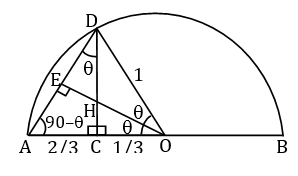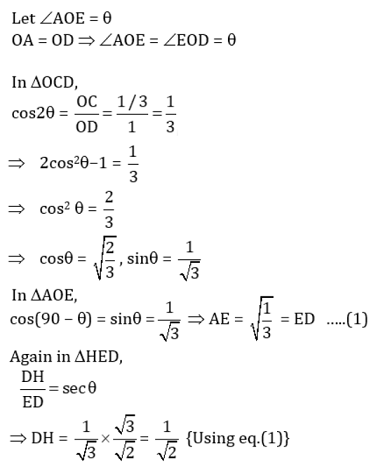Question 19: Let S1 be the sum of areas of the squares whose sides are parallel to coordinate axes. Let S2 be the sum of areas of the slanted squares as shown in the figure. Then S1 / S2 is: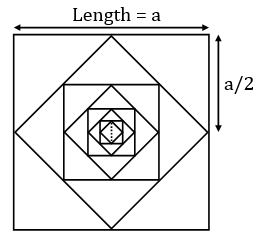1. a. 2
2. b. √2
3. c. 1
4. d. 1/√2

Solution:

S1 = a2 + a2/4 + a2/16 + ….. ∞

{Infinite G.P, First Term = a2, Common ratio = 1/4}

= a2/(1-1/4)

= 4a2/3

S2 = a2/2 + a2/8 + a2/32 + ….. ∞

{Infinite G.P, First Term = a2/2, Common ratio = 1/4}

= (a2/2)/(1-1/4)

= 2a2/3

Therefore, S1/S2 = 2

Question 20: If a 3-digit number is randomly chosen, what is the probability that either the number itself or some permutation of the number (which is a 3-digit number) is divisible by 4 and 5?

1. a. 1/45
2. b. 29/180
3. c. 11/60
4. d. 1/4

Solution:

Here, we are looking for three-digit numbers divisible by 4 and 5 i.e. numbers divisible by 20.

Now, if a number is divisible by 20 it ends with 20, 40, 60, 80, or 100.

(I) The 3-digit numbers ending with 100 are

{100, 200, ………, 900}

i.e. ‘9’ such numbers. ……..(i)

(II) The numbers ending with 20, 40, 60, 80 are

{120, 220……..920,

140, 240……..

160, 260……..

180, ……..980}

i.e. a × 4 = 36 such numbers.

(a) Among above numbers {220, 440, 660, 880} each one of these gives four more numbers after permutation of their digits i.e. {202, 404, 606, 808}

(b) Rest of the numbers give three more numbers after Permutation of their digits

For example, 120 -> 102, 201, 210

Total such numbers = 4 × 2 + 32 × 4

= 8 + 128

= 136 …….. (ii)

From (i) and (ii),

Total number of required numbers = 136 + 9 = 145.

So, the required probability = 45/900 = 29/180.

### KVPY-SA 2016 Maths Paper with Solutions